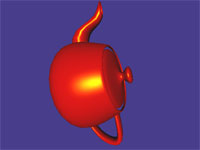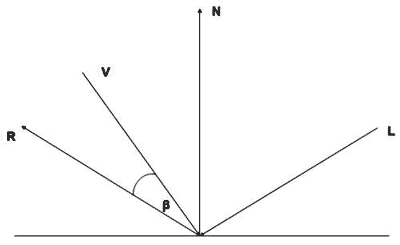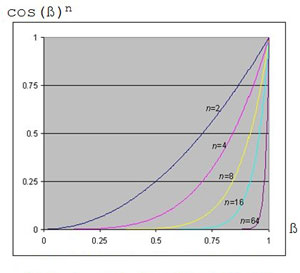## 镜面反射光照

viewMatrix= Matrix.CreateLookAt( new Vector3(x, y, zHeight), Vector3.Zero, Vector3.Up );

"The eye" 的位置在这：

Vector3(x, y, zHeight)

I=AiAc+Di*Dc*N.L+Si*Sc*(R.V)^nfloat4x4 matWorldViewProj;
float4x4 matWorld;
float4 vecLightDir;
float4 vecEye;
float4 vDiffuseColor;
float4 vSpecularColor;
float4 vAmbient;

struct OUT
{
float4 Pos : POSITION;
float3 L : TEXCOORD0;
float3 N : TEXCOORD1;
float3 V : TEXCOORD2;
}; 

float4 PosWorld = mul(Pos,matWorld);
Out.V= vecEye - PosWorld 

OUT VS(float4 Pos : POSITION, float3 N :NORMAL)
{
OUT Out = (OUT)0;
Out.Pos = mul(Pos, matWorldViewProj);
Out.N = mul(N, matWorld);
float4 PosWorld = mul(Pos, matWorld);
Out.L = vecLightDir;
Out.V = vecEye - PosWorld;
return Out;
} 

R = 2 * (N.L) * N – L

float3 Reflect = normalize(2 * Diff * Normal - LightDir);

float3 Reflect = normalize(reflect(-LightDir, Normal));

n表示物体的光泽程度，n越大，反光区域越小。您可能注意到，我们使用了一个新的HLSL函数pow(a,b)，它返回a的b次方。

float Specular = pow(saturate(dot(Reflect, ViewDir)), 15);

return vAmbient + vDiffuseColor * Diff + vSpecularColor * Specular;

float4 PS(float3 L: TEXCOORD0, float3 N : TEXCOORD1, float3 V : TEXCOORD2) : COLOR
{
float3 Normal = normalize(N);
float3 LightDir = normalize(L);
float3 ViewDir = normalize(V);
float Diff = saturate(dot(Normal, LightDir));
// R = 2 * (N.L) * N – L float3
Reflect = normalize(2 * Diff * Normal - LightDir);
float Specular = pow(saturate(dot(Reflect, ViewDir)), 15); // R.V^n
// I = A + Dcolor * Dintensity * N.L + Scolor * Sintensity * (R.V)n
return vAmbient + vDiffuseColor * Diff + vSpecularColor * Specular;
}


technique SpecularLight
{
pass P0
{
}
}

float4x4 matWorldViewProj;
float4x4 matWorld;
float4 vecLightDir;
float4 vecEye;
float4 vDiffuseColor;
float4 vSpecularColor;
float4 vAmbient;
struct OUT
{
float4 Pos : POSITION;
float3 L : TEXCOORD0;
float3 N : TEXCOORD1;
float3 V : TEXCOORD2;
};

OUT VS(float4 Pos : POSITION, float3 N : NORMAL)
{
OUT Out = (OUT)0;
Out.Pos = mul(Pos, matWorldViewProj);
Out.N = mul(N, matWorld);
float4 PosWorld = mul(Pos, matWorld);
Out.L = vecLightDir;
Out.V = vecEye - PosWorld;
return Out;
}
float4 PS(float3 L: TEXCOORD0, float3 N : TEXCOORD1, float3 V : TEXCOORD2) : COLOR
{
float3 Normal = normalize(N);
float3 LightDir = normalize(L);
float3 ViewDir = normalize(V);
float Diff = saturate(dot(Normal, LightDir));
// R = 2 * (N.L) * N - L
float3 Reflect = normalize(2 * Diff * Normal - LightDir);
float Specular = pow(saturate(dot(Reflect, ViewDir)), 15); // R.V^n
// I = A + Dcolor * Dintensity * N.L + Scolor * Sintensity * (R.V)n
return vAmbient + vDiffuseColor * Diff + vSpecularColor * Specular;
}

technique SpecularLight
{
pass P0
{
}
} 

Vector4 vecEye = new Vector4(x, y, zHeight,0);

effect.Parameters["vecEye"].SetValue(vecEye);

## 练习

1． 创建一个新的全局变量指定对象的“shininess”。您可以在应用程序中设定这个值。